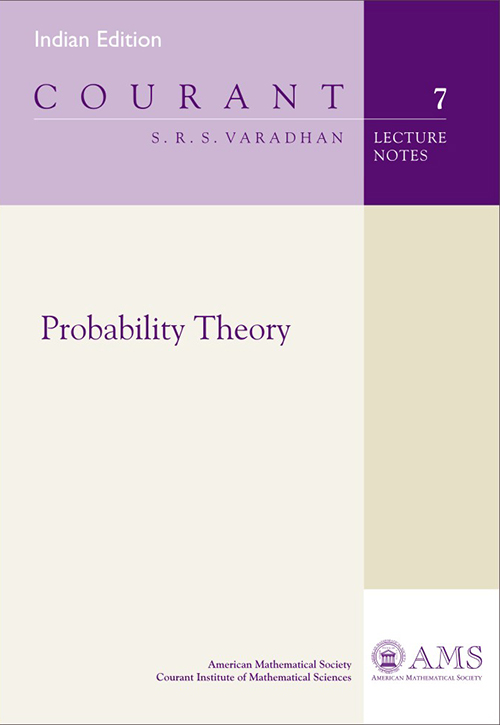Probability Theory
Price
1320.00
ISBN
9781470419141
Language
English
Pages
176
Format
Paperback
Dimensions
180 x 240 mm
Year of Publishing
2014
Territorial Rights
Restricted
Imprint
Universities Press
Catalogues
This volume presents topics in probability theory covered during a first-year graduate course given at the Courant Institute of Mathematical Sciences. The necessary background material in measure theory is developed, including the standard topics, such as extension theorem, construction of measures, integration, product spaces, Radon-Nikodym theorem, and conditional expectation. In the first part of the book, characteristic functions are introduced, followed by the study of weak convergence of probability distributions.

Then both the weak and strong limit theorems for sums of independent random variables are proved, including the weak and strong laws of large numbers, central limit theorems, laws of the iterated logarithm, and the Kolmogorov three series theorem. The first part concludes with infinitely divisible distributions and limit theorems for sums of uniformly infinitesimal independent random variables.

The second part of the book mainly deals with dependent random variables, particularly martingales and Markov chains. Topics include standard results regarding discrete parameter martingales and Doob's inequalities. The standard topics in Markov chains are treated, i.e., transience, and null and positive recurrence. A varied collection of examples is given to demonstrate the connection between martingales and Markov chains.

Additional topics covered in the book include stationary Gaussian processes, ergodic theorems, dynamic programming, optimal stopping, and filtering. A large number of examples and exercises is included. The book is a suitable text for a first-year graduate course in probability.
Preface vii
Chapter 1. Measure Theory 1
1.1. Introduction 1
1.2. Construction of Measures 3
1.3. Integration 7
1.4. Transformations 13
1.5. Product Spaces 14
1.6. Distributions and Expectations 16

Chapter 2. Weak Convergence 19
2.1. Characteristic Functions 19
2.2. Moment-Generating Functions 22
2.3. Weak Convergence 24

Chapter 3. Independent Sums 35
3.1. Independence and Convolution 35
3.2. Weak Law of Large Numbers 37
3.3. Strong Limit Theorems 40
3.4. Series of Independent Random Variables 43
3.5. Strong Law of Large Numbers 48
3.6. Central Limit Theorem 49
3.7. Accompanying Laws 54
3.8. Infinitely Divisible Distributions 59
3.9. Laws of the Iterated Logarithm 66

Chapter 4. Dependent Random Variables 73
4.1. Conditioning 73
4.2. Conditional Expectation 79
4.3. Conditional Probability 81
4.4. Markov Chains 84
4.5. Stopping Times and Renewal Times 89
4.6. Countable State Space 90
4.7. Some Examples 98
Chapter 5. Martingales 109
5.1. Definitions and Properties 109
5.2. Martingale Convergence Theorems 112

v
CONTENTS
5.3. Doob Decomposition Theorem 115
5.4. Stopping Times 117
5.5. Up-crossing Inequality 120
5.6. Martingale Transforms, Option Pricing 121
5.7. Martingales and Markov Chains 123

Chapter 6. Stationary Stochastic Processes 131
6.1. Ergodic Theorems 131
6.2. Structure of Stationary Measures 135
6.3. Stationary Markov Processes 137
6.4. Mixing Properties of Markov Processes 141
6.5. Central Limit Theorem for Martingales 143
6.6. Stationary Gaussian Processes 147

Chapter 7. Dynamic Programming and Filtering 157
7.1. Optimal Control 157
7.2. Optimal Stopping 158
7.3. Filtering 161

Bibliography 163
Index 165
THE BOOKPOINT (INDIA) PVT. LTD.